# Learn C language - Tutorial 3 : How to add two numbers in CAddition of number program is the basic program in C or any other language.
In the first C tutorial I gave the basic C code to display “This is my first C program”.
In this post i will give the tips to add numbers in C program.
There are two types of implementing this program.
In the first method We can add the numbers by declaring the values at the programming time itself this method is called as “Static method”.
Let we see how to add the numbers using this method.
Type the following code in the C compiler for example Turbo c++
#include<stdio.h>
#include<conio.h>
void main()
{
int a=5,b=3,c;
clrscr();
c=a+b;
getch();
}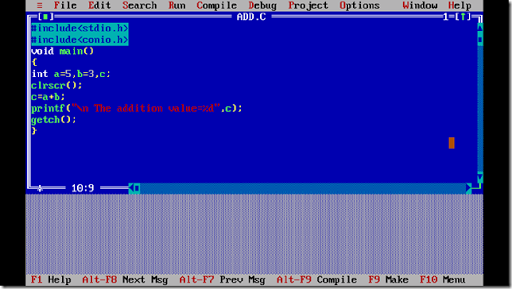After typing the code press F2 to save and save it as add.c the saving syntax for c is filename.c
Then we need to compile the program using ALT+F9.
Then run the program by pressing CTRL+F9
You can see the output below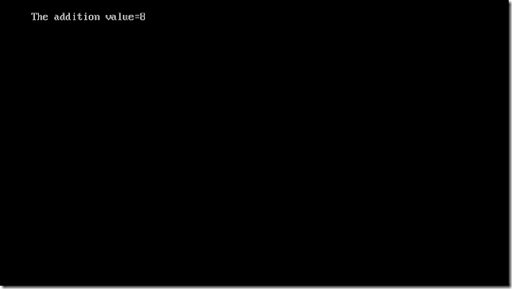You can see that the addition value of5 and 3 is 8.
PROGRAM EXPLANATION
The first two lines are called as Header Files
The first line of the program is
#include<stdio.h>
Stdio stands for Standard Input and Output ,
this is used to get the input from the show the output to the user by using predefined(already present in the C library) functions(it is nothing but it do the some task in this case input and output) for example: printf for printing the output in the screen, scanf for getting input from the user.
The second line is
#include<conio.h>
This is Console input and output it is used for connecting the consoles for example: clrscr() this is used to clear the screen of the monitor to erase the previous output of the program you have done before.
the next is
void main()
This is the main function from where the actual programming starts.
void is a return type which returns nothing but it mostly used in order to avoid some errors.
the next is
{
}
this denotes that the coding must be inside this braces.
The next line is
int a=5,b=3,c;
The above line is called as declaration statement(it means saying to the program that i am a part of this program with the certain values).
int is called as Data Type.
Data Type means the type of the data , in the case of addition program we use the data of integer (number) types thus we are using int data type , if we operate on names we use char data type (i.e character ).
Next the a,b,c are called as variables.
Variables are nothing but creating a storage space for the values to be stored, since we are storing multiple values we use multiple variables.
In the above program we used static method so we have declared the values for the variables.
The next line is clrscr() , this is a function that is used to clear the output screen.
The next line is
c=a+b;
The next line in the program is
printf() is the function that is used to print the output on the screen.
the output to be displayed is written within the double quotes “ ”
the %d is for printing the integer value and the c is for printing the addition value stored in the variable c.
the next line is
getch() function is used to print the output stable, if you are not putting this line the compiler will not show the error but the output will not be displayed thus this is very important.
If you notice at the end of each line i have put semi colon ; it indicates that end of the statement.
I hope you have understand the basic concepts in programming.
Let we go to the next method of addition is by getting the input of the two variables from the user
Type the code in the Turbo c++
#include<stdio.h>
#include<conio.h>
void main()
{
int a,b,c;
clrscr();
printf(“\n enter the value of a:”);
scanf(“%d”,&a);
printf(“\n enter the value of b:”);
scanf(“%d”,&b);
c=a+b;
getch();
}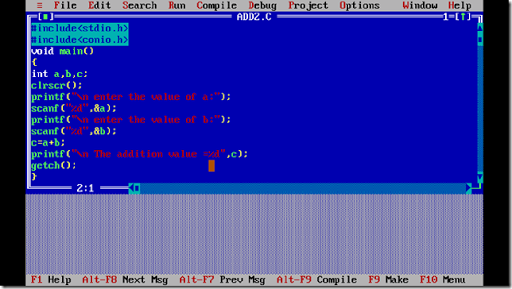Then compile the program and run the program
First it will ask for the value of a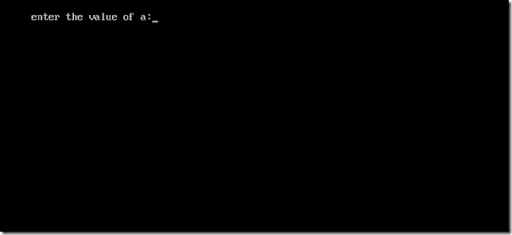Enter the value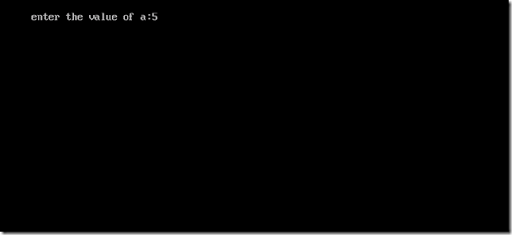Then it will ask for the value of b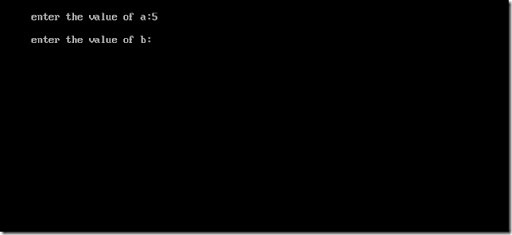Then give the value for the variable b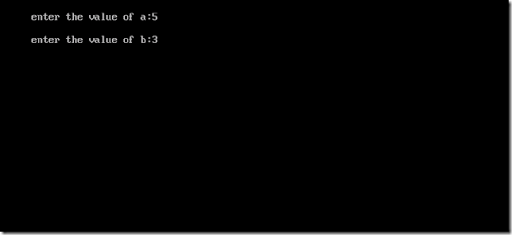Then the result will be displayed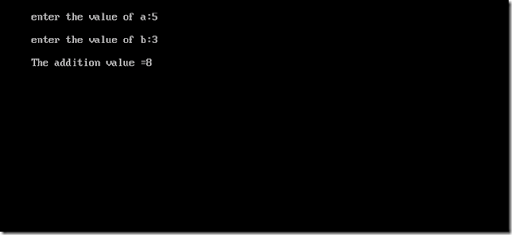That’s it we have written the program to add two numbers in two different ways…
Hope the tip was useful…